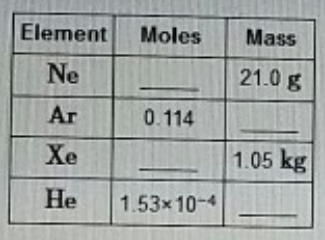# Problem: a) Complete the second column of the table. b) Complete the third column of the table.

###### FREE Expert Solution###### Problem Details

a) Complete the second column of the table.

b) Complete the third column of the table.What scientific concept do you need to know in order to solve this problem?

Our tutors have indicated that to solve this problem you will need to apply the Mole Concept concept. You can view video lessons to learn Mole Concept. Or if you need more Mole Concept practice, you can also practice Mole Concept practice problems.

What is the difficulty of this problem?

Our tutors rated the difficulty ofa) Complete the second column of the table. b) Complete the ...as low difficulty.

How long does this problem take to solve?

Our expert Chemistry tutor, Sabrina took 4 minutes and 38 seconds to solve this problem. You can follow their steps in the video explanation above.

What professor is this problem relevant for?

Based on our data, we think this problem is relevant for Professor Rivas' class at MDC.